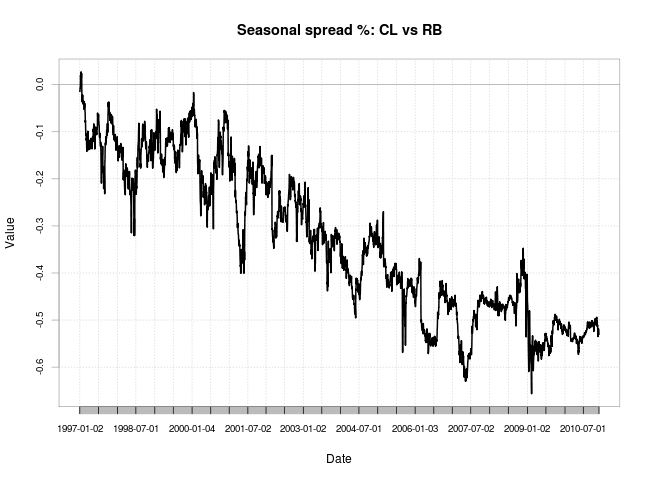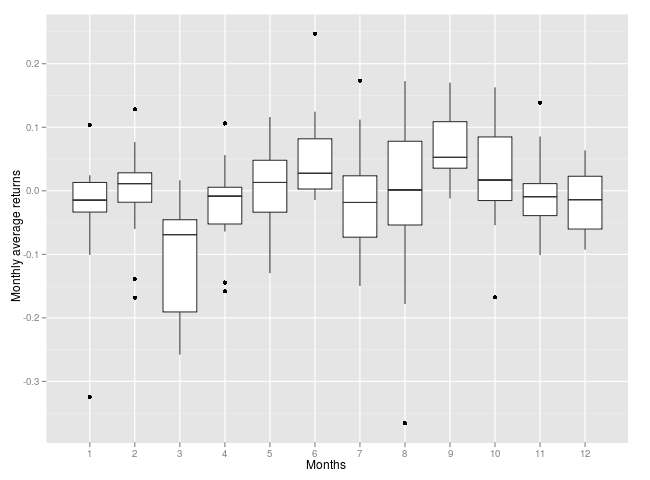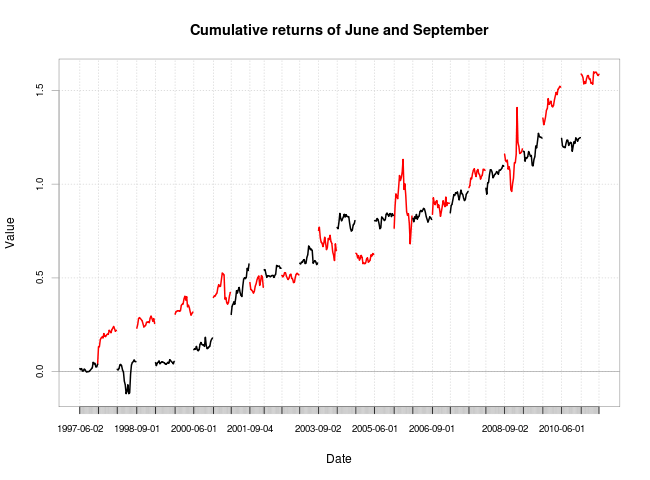[This article was first published on Quantitative thoughts » EN, and kindly contributed to R-bloggers]. (You can report issue about the content on this page here)
Want to share your content on R-bloggers? click here if you have a blog, or here if you don't.

quanttrader.info is a good quantitative repository, where I found an idea about seasonal spreads play.

The idea of seasonal pair trading differs from pairs trading in a way, that it doesn’t try to find deviation from the spread’s mean, but it looks at seasonal spread patterns. In some cases it is easier to find an explanation, why seasonal spread works at all. For example, during the winter time the consumption of heating oil goes up, but it is opposite for gasoline. During the summer is just opposite – because of holidays the demand for gasoline shuts up.

The data

Be aware, that you can obtain different results, because a lot of depends on the data quality and understanding of the data. In real world continuous contract doesn’t exist. Yes, there is some shops/brokers which provide such contract, but NYMEX exchange has future contracts with fix duration. In later case, you have to derive your own continuous contract and revolve it each month in case it is front month contract.

To run this test, I took the data from here. I had to relay on freely available data in this case, because I don’t have access to commercial data for such long period. Let me know, if have substantial differences in the result of this test with others data providers. Here is some differences between my results and the results share by quanttrader.info.

The test

First of all, let’s plot cumulative returns of the oil (CL) and the gasoline (RB) front month contracts:The next graph shows cumulative spread between CL and RB in percentage terms. It is difficult to spot any seasonal pattern just by looking at it, except that during some years it was trending down. This can be a problem for long term investment (let say more that 3 months – it is just an educated guess).Let’s look what are daily averages aggregated by month in percentage terms:

01 -0.12%
02 0.01%
03 -0.44%
04 -0.08%
05 0.02%
06 0.28%
07 -0.04%
08 -0.03%
09 0.348%
10 0.12%
11 -0.009%
12 -0.07%
As we can see, here is 3 months (in bold), which have average returns deviated from its daily mean -0.0029%. Because averages can be misleading, it is worth to check intervals of these averages. But this time, instead of daily means I used monthly returns to generate following graph:The graph above shows, that some months had the returns around zero or the returns were distributed very wildly, for example like August. However, during March, June and September the returns were very consistent. Let’s take a look on March’s cumulative return:Here is the problem – during the last years the curve flattened and March’s returns are close to zero.Well, it basically means, that you have to avoid investing in spread during this month.

Now, let’s check what were the cumulative returns of June (black) and September (red)?This time the returns are much more consistent and can be used for further development.

The final word

The results of my study do not support the result obtained by Paul Teetor. Most likely the differences come from the data. I used free data and I can’t be sure, that this data repository can be trusted. In this study I used front month contracts, which are expiring in the same month. If you try the same study with the following month, then results will be different as well.

Paul Teetor mentioned in his study, that he prefers to deal with dolor returns, however my study is based on price returns. I tried to obtain the hedge value 1.13 disclosed in his study, but I got it my way as presented below. The hedge value is important, because you have to know how much invest in each asset. The reason for that is, that each asset can have different volatility and you need different amount of money for short leg and another amount for long leg. Below is the graph where you can see yearly difference between volatilities of Cl and RB:When the value is above zero, then you have underweight oil and overweight gasoline, because the latter is less volatile. By the way, this graph doesn’t provide the hedge ratio – it is just proof of concept.

The source file the can be find on github or by clicking on View Code below.

?View Code RSPLUS
 ```require('xts') require('quantmod') Sys.setenv(TZ="GMT")   require('PerformanceAnalytics')   tmp<-as.matrix(read.table('tickers/various_day_close/rb_contract1.csv',sep=',',header=FALSE)) rb<-as.xts(as.double(tmp[,2]),order.by=as.POSIXct(strptime(tmp[,1],'%Y-%m-%d')))   rb<-tail(rb,-3)['::2010-11']     tmp<-as.matrix(read.table('tickers/various_day_close/cl_contract1.csv',sep=',',header=FALSE)) cl<-as.xts(as.double(tmp[,2]),order.by=as.POSIXct(strptime(tmp[,1],'%Y-%m-%d'))) cl<-tail(cl,-3)['::2010-11']     rb.delta<-Delt(((rb)))['1997-01::'] cl.delta<-Delt(((cl)))['1997-01::']   rb.delta[is.na(rb.delta)]<-0 cl.delta[is.na(cl.delta)]<-0   spread<-cl.delta*50000-rb.delta*50000   png('spread_cl_prices.png',width=650) chart.CumReturns(cbind(cl.delta,rb.delta),col=c(2,3),main='Oil & Gasoline prices') dev.off()   png('spread_cl_rb.png',width=650) chart.TimeSeries(cumsum(spread),main='Seasonal spread: CL vs RB') dev.off()   spread<-cl.delta-rb.delta   png('spread_cl_rb_prc.png',width=650) chart.CumReturns((spread),main='Seasonal spread %: CL vs RB') dev.off()   spread.factor<-as.factor(format(index(spread),'%m')) aggregate(spread, spread.factor,mean) summary(lm(as.double(spread)~(spread.factor)))       rb.delta.monthly<-Delt(Cl(to.monthly(rb)))['1997-01::'] cl.delta.monthly<-Delt(Cl(to.monthly(cl)))['1997-01::']   rb.delta.monthly[is.na(rb.delta.monthly)]<-0 cl.delta.monthly[is.na(cl.delta.monthly)]<-0   factor<-as.factor(format(index(cl.delta.monthly-rb.delta.monthly),'%m')) tmp<-data.frame(as.double(cl.delta.monthly-rb.delta.monthly),as.numeric(factor))   require('ggplot2') png('monthly_averages.png',width=650) qplot(factor(as.numeric(factor)),as.double(cl.delta.monthly-rb.delta.monthly),data=tmp,geom = "boxplot",ylab='Monthly average returns',xlab='Months') dev.off()   png('march_cumulative.png',width=650) chart.CumReturns(spread[spread.factor=='03'],main='March cumulative return') dev.off()   png('yearly_diff.png',width=650) chart.TimeSeries(cbind(as.xts(rollapply(rb.delta,250,sd,align='right'))-as.xts(rollapply(cl.delta,250,sd,align='right'))),main='Yearly difference of vol. between CL & RB') dev.off()```

To leave a comment for the author, please follow the link and comment on their blog: Quantitative thoughts » EN.

R-bloggers.com offers daily e-mail updates about R news and tutorials about learning R and many other topics. Click here if you're looking to post or find an R/data-science job.
Want to share your content on R-bloggers? click here if you have a blog, or here if you don't.

# Never miss an update! Subscribe to R-bloggers to receive e-mails with the latest R posts.(You will not see this message again.)

Click here to close (This popup will not appear again)